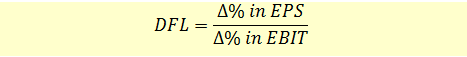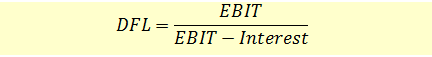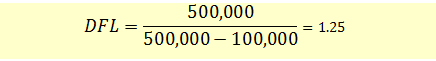# Degree of Financial Leverage

Financial leverage arises when a firm has fixed financing costs in its income stream. The presence of financial leverage magnifies the effects of changes in sales to the firm’s net earnings. Sources of financial leverage are primarily debt and preferred stock. The British expression for financial leverage is gearing.

The degree of financial leverage is a leverage ratio that measures the percentage change in a firm’s earnings per share (EPS) resulting from a 1% change in operating profit.We can calculate the DFL of a company at a particular level of operating earnings as follows:Annie Davis wants to determine the degree of financial leverage at earnings level of $500,000. Assuming that the interest expense is$100,000, the degree of financial leverage will be calculated as follows:If debt increases 1%, the earnings per share will increase by 1.25%.

Note that as the fixed financing costs increase, the DFL will increase. DFL will be equal to 1 when there is no fixed financing cost. For both DFL and DOL, a value of 1 means that there is no leverage.